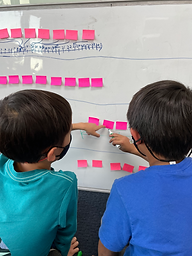Ms. Caitlyn

Target 1​

Lesson Type:

New

Number Operation

:

Fractional Numbers

Identify, compare, and manipulate different types of decimals.

1:

Understand that decimals represent quantities that have a portion that is less than a whole.

2:

Read and write numbers in decimal notation.

3:

Place decimals on a number line.

4:

Understand that decimals on the number line are smaller farther to the left and greater farther to the right.

5th

Vocabulary:

Decimal Notation, Tenths, Hundredths, Thousandths

Activities:

• Students placed decimals from least to greatest.
• Students organized sets of decimals on a number line.
• Students rewrote words - such as six and ten thousandths - as a decimal (6.010).
• Students listened to the teacher read decimals out loud and marked them off on their Bingo boards.Home Exploration

Guiding Questions:Absent Students:

Target 2

:

1:

Understand equivalent measurements.

2:

Understand how to convert from one unit to another.

5th

Vocabulary:

U.S. Customary System, Metric System

Activities:

• Students solved task cards involving unit conversion and measurement-related word problems.Home Exploration

Guiding Questions:Target 3

:

Vocabulary:

Activities:Home Exploration